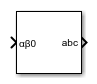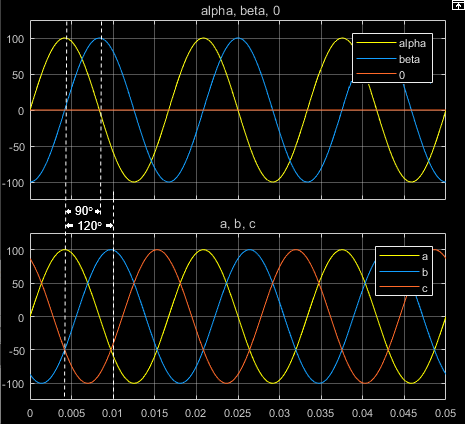# Inverse Clarke Transform

Implement αβ0 to abc transform

•Libraries:
Simscape / Electrical / Control / Mathematical Transforms

## Description

The Inverse Clarke Transform block converts the time-domain alpha, beta, and zero components in a stationary reference frame to three-phase components in an abc reference frame. The block can preserve the active and reactive powers with the powers of the system in the stationary reference frame by implementing an invariant power version of the inverse Clarke transform. If the zero component is zero, the components in the three-phase system are balanced.

The figures show:

• Balanced ɑ, β, and zero components in a stationary reference frame• The direction of the magnetic axes of the stator windings in the stationary ɑβ0 reference frame and the abc reference frame• The time-response of the individual components of equivalent balanced ɑβ0 and abc systems### Equations

The block implements the inverse Clarke transform as

`$\left[\begin{array}{c}a\\ b\\ c\end{array}\right]=\left[\begin{array}{ccc}1& 0& 1\\ -\frac{1}{2}& \frac{\sqrt{3}}{2}& 1\\ -\frac{1}{2}& -\frac{\sqrt{3}}{2}& 1\end{array}\right]\left[\begin{array}{c}\alpha \\ \beta \\ 0\end{array}\right]$`

where:

• α and β are the components in the stationary reference frame.

• 0 is the zero component in the stationary reference frame.

• a, b, and c are the components of the three-phase system in the abc reference frame.

The block implements this power invariant version of the inverse Clarke transform as

`$\left[\begin{array}{c}a\\ b\\ c\end{array}\right]=\sqrt{\frac{2}{3}}\left[\begin{array}{ccc}1& 0& \frac{1}{\sqrt{2}}\\ -\frac{1}{2}& \frac{\sqrt{3}}{2}& \frac{1}{\sqrt{2}}\\ -\frac{1}{2}& -\frac{\sqrt{3}}{2}& \frac{1}{\sqrt{2}}\end{array}\right]\left[\begin{array}{c}\alpha \\ \beta \\ 0\end{array}\right]$`

## Ports

### Input

expand all

Alpha-axis component,α, beta-axis component β, and zero component in the stationary reference frame.

Data Types: `single` | `double`

### Output

expand all

Components of the three-phase system in the abc reference frame.

Data Types: `single` | `double`

## Parameters

expand all

Preserve the active and reactive power of the system in the rotating reference frame.

 Krause, P., O. Wasynczuk, S. D. Sudhoff, and S. Pekarek. Analysis of Electric Machinery and Drive Systems. Piscatawy, NJ: Wiley-IEEE Press, 2013.